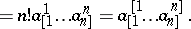# Alternation

skew symmetry, anti-symmetry, alternance

One of the operations of tensor algebra, yielding a tensor that is skew-symmetric (over a group of indices) from a given tensor. Alternation is always effected over a few superscripts or over a few subscripts. A tensorwith components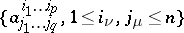is the result of alternation of a tensorwith components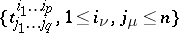, for example, over superscripts, over a group of indices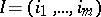if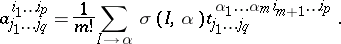(*)

The summation is conducted over all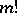rearrangements (permutations)of, the numberbeingor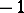, depending on whether the respective rearrangement is even or odd. Alternation over a group of subscripts is defined in a similar manner.

Alternation over a group of indices is denoted by enclosing the indices between square brackets. Secondary indices inside the square brackets are separated by vertical strokes. For instance: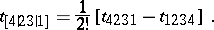Successive alternation over groups of indices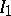and,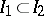, coincides with alternation over the group of indices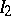: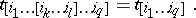Ifis the dimension of the vector space on which the tensor is defined, alternation by a group of indices the number of which is larger thanwill always produce the zero tensor. Alternation over a given group of indices of a tensor which is symmetric with respect to this group (cf. Symmetrization (of tensors)) also yields the zero tensor. A tensor that remains unchanged under alternation over a given group of indicesis called skew-symmetric or alternating over. Interchanging any pair of such indices changes the sign of the component of the tensor.

The operation of tensor alternation, together with the operation of symmetrization, is employed to decompose a tensor into simpler tensors.

The product of two tensors with subsequent alternation over all indices is called an alternated product (exterior product).

Alternation is also employed to produce sign-alternating sums of the form (*) with multi-indexed terms. For instance, a determinant with elements which commute under multiplication can be computed by the formulas